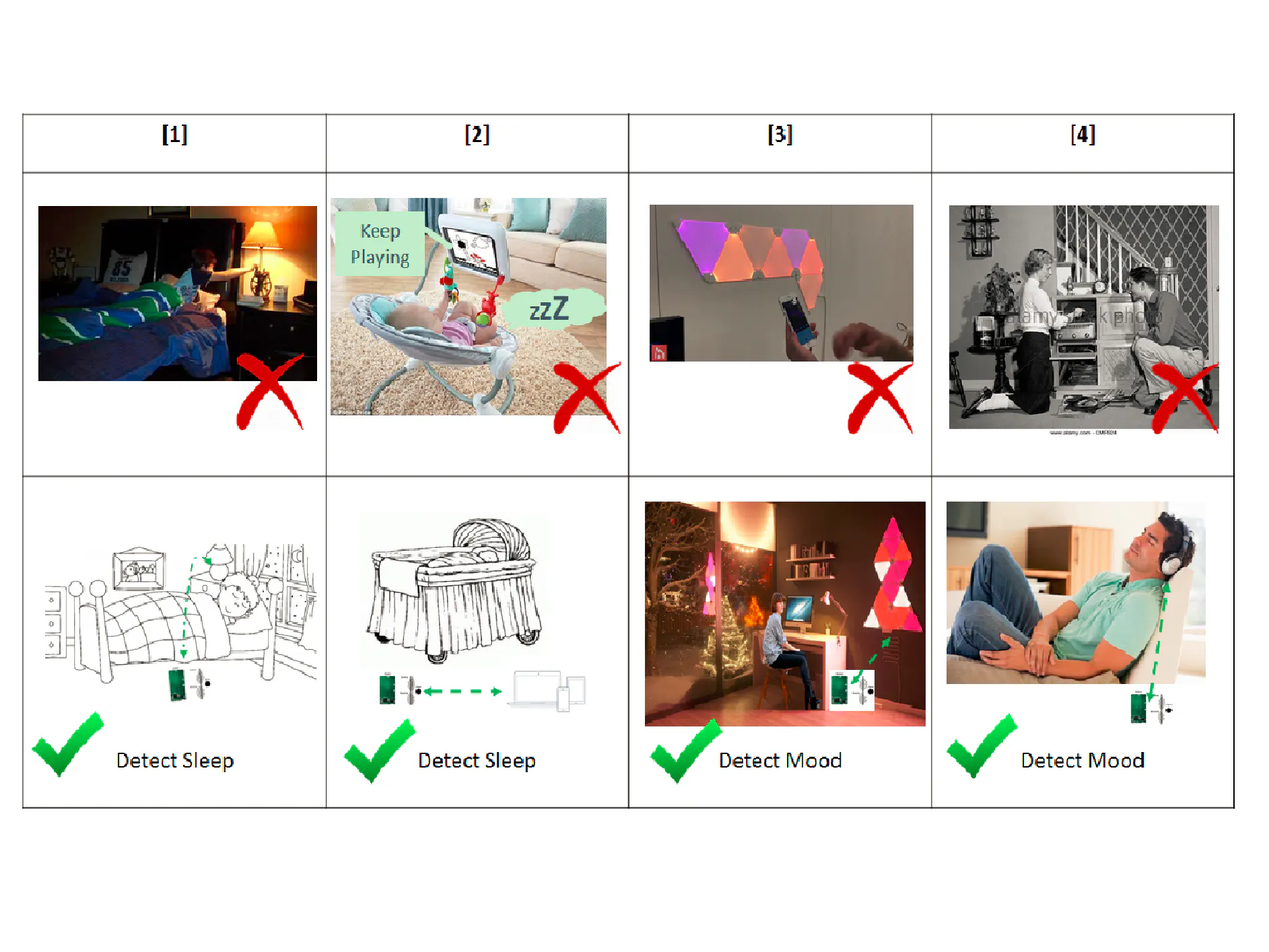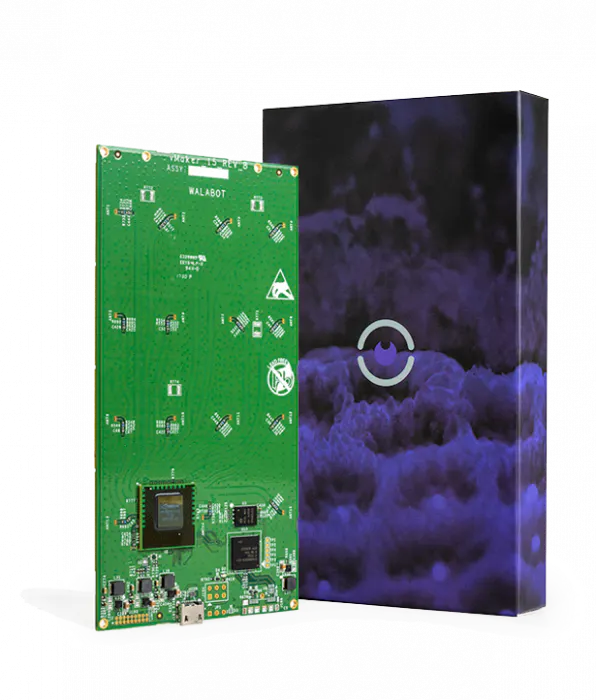# Sleeping and Mood Switch

This project is creating an intelligent switch connecting people's sleep to the switchers button of any type of intelligence devices.

BeginnerFull instructions provided2 hours1,246## Things used in this project

### Hardware componentsWalabot Creator
×1Raspberry Pi 3 Model B
×1Jumper wires (generic)
×1LED (generic)
×1ControlEverything.com Relay Shield for Particle Photon I²C 8-Channel SPDT 10-Amp
×1

### Software apps and online servicesMicrosoft Windows 10 IoT Core
 PuTTy We are going to need to be able to connect to our Raspberry Pi via SSH. So PuTTY is the perfect client to do this.

## Code

### Sleep_Switch_v1.0.4

Python
1) A light controller based on breath detection by Walabot.
2) Screen output to write to txt file, so could collect and analysis data
3) Implemented with a timer to control circle test print every 5s and judge every 10s
4) sleeping criteria in: mean < 0.02, max <0.02, min <0.01, std <0.01
```from __future__ import print_function
from sys import platform
from os import system
import WalabotAPI as wlbt
import time # python timer
import statistics #cal std
import RPi.GPIO as GPIO #This imports the GPIO Library into Python so we can use the GPIO Pins on the Pi.

GPIO.setmode(GPIO.BCM)
GPIO.setup(2, GPIO.OUT) #We are using GPIO 2 as our first pin, which is where the green wire is connected to the first relay switch on the baord
#initialize as false, thus off

wlbt.Init()  # load the WalabotSDK to the Python wrapper
wlbt.SetSettingsFolder()  # set the path to the essetial database files
wlbt.ConnectAny()  # establishes communication with the Walabot

wlbt.SetProfile(wlbt.PROF_SENSOR_NARROW)  # set scan profile out of the possibilities
# JC: replace PROF_SENSOR to PROF_SENSOR_NARROW
# Sensor narrow: Lower-resolution images for a fast capture rate. Useful for tracking quick movement.
# thus for heart rate
wlbt.SetDynamicImageFilter(wlbt.FILTER_TYPE_DERIVATIVE)  # specify filter to use
#JC: replace FILTER_TYPE_MTI to FILTER_TYPE_DERIVATIVE
#Moving Target Identification (MTI) filter, the Derivative filter is available for the specific frequencies typical of breathing.

## variables definition
## Walabot_SetArenaR - input parameters
minInCm = 30;
maxInCm = 150;
resICm = 1;
## Walabot_SetArenaTheta - input parameters
minIndegrees = -4;
maxIndegrees = 4;
resIndegrees = 2;
## Walabot_SetArenaPhi - input parameters
minPhiInDegrees = -4;
maxPhiInDegrees = 4;
resPhiInDegrees = 2;
##JC: here below modified from C++ breathing code, before use variables needs to be defined,  parameters accoarding to http://api.walabot.com/_features.html and http://api.walabot.com/_sample.html C++ breathing code
## Setup arena - specify it by Cartesian coordinates(ranges and resolution on the x, y, z axes);
##  In Sensor mode there is need to specify Spherical coordinates(ranges and resolution along radial distance and Theta and Phi angles).
wlbt.SetArenaR(minInCm, maxInCm, resICm)
## Sets polar range and resolution of arena (parameters in degrees).
wlbt.SetArenaTheta(minIndegrees, maxIndegrees, resIndegrees)
## Sets azimuth range and resolution of arena.(parameters in degrees).
wlbt.SetArenaPhi(minPhiInDegrees, maxPhiInDegrees, resPhiInDegrees)

wlbt.Start()  # starts Walabot in preparation for scanning
print ('hello')
thefile = open('%s.txt' % time.strftime("%H-%M-%S_%d-%m-%Y"), 'w') #open file with name of current time

while True:
MEAN = [] #array which save each mean get from wlbt
MAX = [] #array which save each max get from wlbt
MIN = [] #array which save each min get from wlbt
STD = [] #array which save each std get from wlbt
start_out = time.time() #timer control how long each circle
while (time.time()-start_out) <10:		#each 1min
Energy = [] #array which save each energy get from wlbt
start_in = time.time() #timer control how long each circle
while (time.time()-start_in) <5:		#each 10s
##JC: add calibrates based from C++ code
##// calibrates scanning to ignore or reduce the signals
wlbt.StartCalibration() #start calibration
while wlbt.GetStatus() == wlbt.STATUS_CALIBRATING: #when calibraion done
wlbt.Trigger() #trigger walabot
Energy.append(wlbt.GetImageEnergy())  # get Energy
#Energy_current = wlbt.GetImageEnergy()
##JC: replace GetSensorTargets to GetImageEnergy
#system('cls' if platform == 'win32' else 'clear')  # clear the terminal
#print('Energy_Current = ', Energy_current, '/n')

#after each 10s do following
print('mean(10s)=', sum(Energy) / len(Energy), ' ')
print('max(10s)=', max(Energy) , ' ')
print('min(10s)=', min(Energy) , ' ')
print('std(10s)=', statistics.stdev(Energy) , '\n')
#print('time elapsed', time.time()-start, ' ' )
MEAN.append(sum(Energy) / len(Energy))  # get Energy
MAX.append(max(Energy))  # get Energy
MIN.append(min(Energy))  # get Energy
STD.append(statistics.stdev(Energy))  # get Energy

thefile.write("%s " % time.strftime("%H:%M:%S"))
for item in Energy:
thefile.write("%s " % item)
thefile.write("\n")

#fed analyzed sleeping criteria in: mean < 0.02, max <0.02, min <0.01, std <0.01
if ((sum(map(abs, MEAN)) / len(MEAN))<0.02): #use absolute
if ((sum(map(abs, MAX)) / len(MAX))<0.02):
if ((sum(map(abs, MIN)) / len(MIN))<0.01):
if ((sum(map(abs, STD)) / len(STD))<0.01):
print ("SLEEP!!!SLEEP!!!SLEEP!!!")
GPIO.output(2, False) #light off
thefile.write(" SLEEP!!!")
thefile.write("\n")
else:
print ("AWAKE")
GPIO.output(2, True)  #light on
thefile.write(" AWAKE!!!")
thefile.write("\n")
else:
print ("AWAKE")
GPIO.output(2, True)  #light on
thefile.write(" AWAKE!!!")
thefile.write("\n")
else:
print ("AWAKE")
GPIO.output(2, True)  #light on
thefile.write(" AWAKE!!!")
thefile.write("\n")
else:
print ("AWAKE")
GPIO.output(2, True)  #light on
thefile.write(" AWAKE!!!")
thefile.write("\n")

wlbt.Stop()  # stops Walabot when finished scanning
wlbt.Disconnect()  # stops communication with Walabot
```

## Credits

### CHEN JIN

1 project • 2 followers
Hi, I am currently a Research Associate on the subject of Computational Geoscience. I take developing as hobbies since end of 2016.